# Basic Geometry Vocabulary Flashcards

Set Details Share
created 2 years ago by amalone
42 views
Subjects:
math, geometry
Page to share:
Embed this setcancel
COPY
code changes based on your size selection
Size:
X
1

Polygon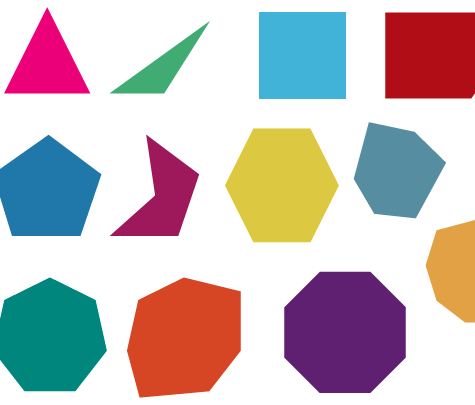Closed figure with straight sides

2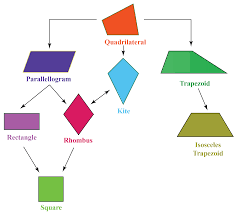4- sided polygon

3

Trapezoid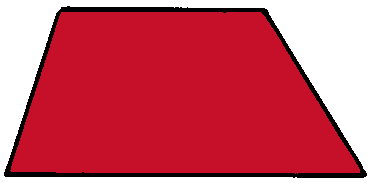A quadrilateral with only one set of parallel lines

4

Parallelogram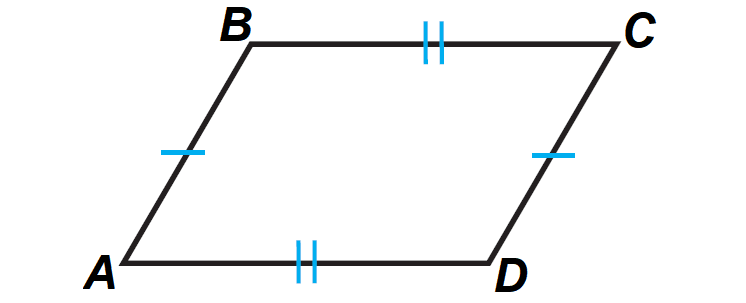A quadrilateral with two sets of parallel lines

5

RhombusA parallelogram (two sets of parallel sides) with all sides the same length

6

RectangleA parallelogram (two sets of parallel sides) with four right angles, opposite sides are of equal length

7

SquareA rhombus (2 sets of parallel lines, all sides of equal length) with four right angles

8

Acute Triangle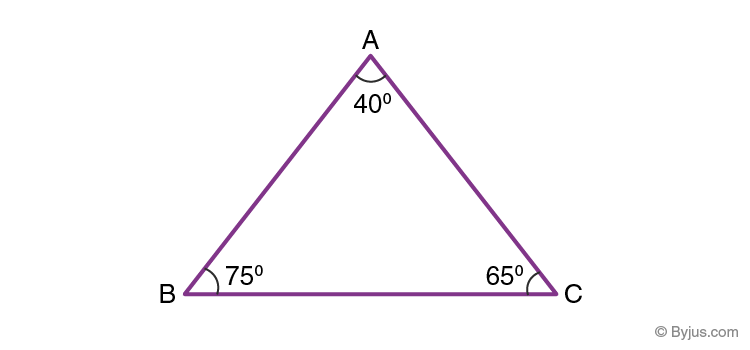A triangle that has all angles less than 90° (90° is a Right Angle)

9

Right Triangle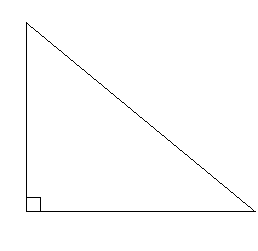A triangle that has a right angle (90°)

10

Obtuse Triangle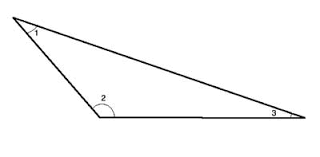A triangle that has an angle greater than 90°

11

Equilateral Triangle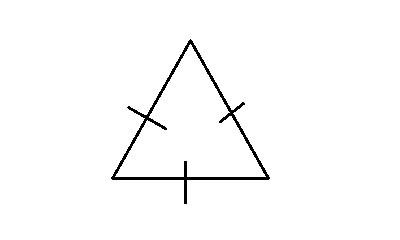A triangle with all three sides of equal length.

All angles are acute.

12

Isosceles TriangleA triangle with two equal sides.

The angles opposite the equal sides are also equal.

13

Scalene Triangle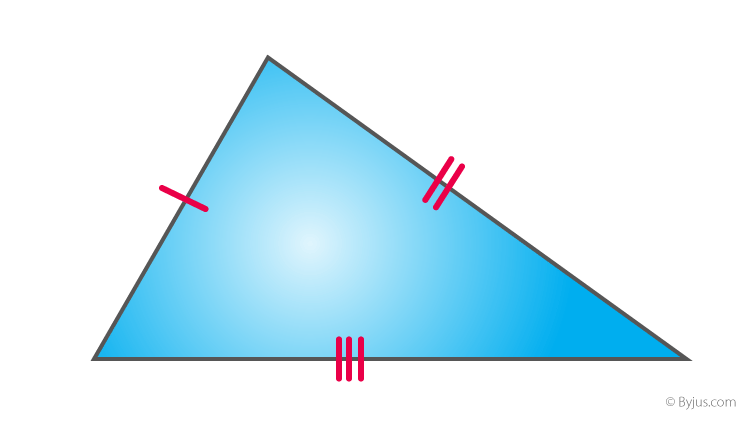A triangle with all sides of different lengths.
All angles are different, too.

14

Pentagon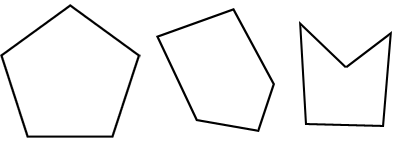5-sided polygon

15

Heptagon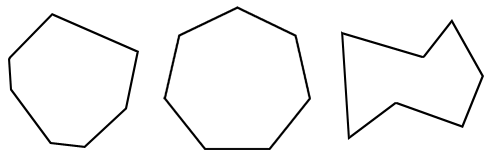7-sided polygon

16

Hexagon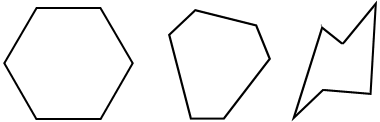6-sided polygon

17

Octagon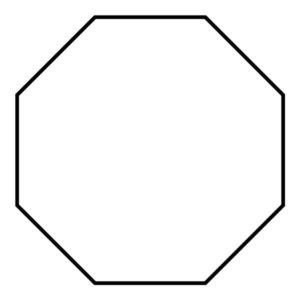8-sided polygon

18

Decagon10- sided polygon